# “If, Else” Formula in Excel with 4 Examples (Very Easy)

The Formula “If” “Else” is very important in excel, with the help of If-Else we can do filter anything easily in excel, so here in this article we will tell you some example of If-Else formula. So let started with example as given here under.

# Example No. 1 for “If- Else” Formula in Excel :

We are starting with very easy example, here in Cell A2 We will enter a short name of day and in Cell B2 we will get the full name of that Day with the help of if-else function.

So type the formula in cell “B2” as given here under:

=IF(A2=”Su”,”Sunday”,IF(A2=”Mo”,”Monday”,IF(A2=”Tu”,”Tuesday”,IF(A2=”We”, “Wednesday”,IF(A2=”Th”,”Thursday”,IF(A2=”Fr”,”Friday”,IF(A2=”Sa”,”Saturday”,)))))))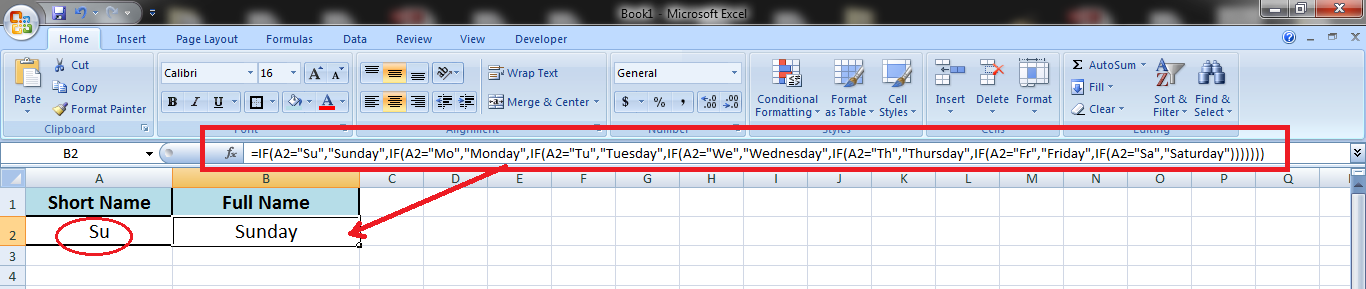Now when we will Enter “Mo” in column A2, we will get the result “Monday”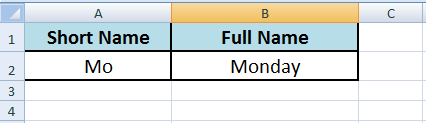Now when we will Enter “Tu” in column A2, we will get the result “Tuesday”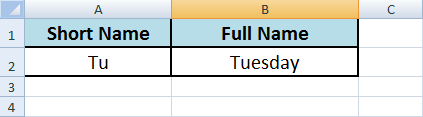Now when we will Enter “We” in column A2, we will get the result “Wednesday”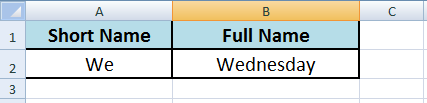Now when we will Enter “Th” in column A2, we will get the result “Thursday”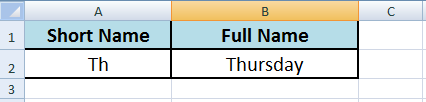Now when we will Enter “Fr” in column A2, we will get the result “Friday”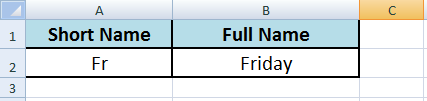Now when we will Enter “Sa” in column A2, we will get the result “Saturday”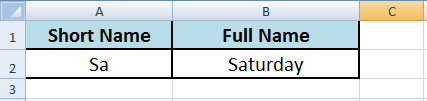# Example No. 2 for “If- Else” Formula in Excel :

Now we are taking another example to understand the use of if-else formula.

If Column “A2” is Blank then Show the Result that “No Data Found”

If Column “A2” contain any amount then Show that value in Column “C2”

So friends enter the below formula in Column no. “C2”

=IF(A2=””,”No Data Found”,A2)

Result will be as under if Cell A2 is Blank-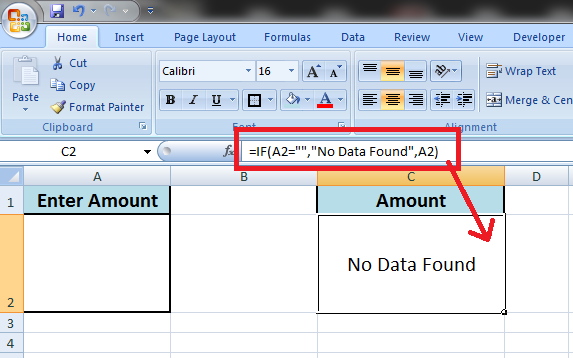And Result will be as under if Cell A2 has a value or amount-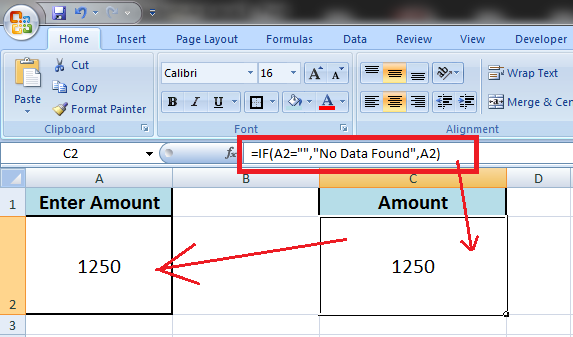# Example No. 3 for “If- Else” Formula in Excel :

Now we are taking another example in which excel sheet contain a mark sheet of an student, now we will set the formula that if Total marks is below or equal  to 150  than student is “Fail” and if Total Marks is above 150 then student is “Passed”.

So we will set the below formula in cell no. “B9” to get the result in “B9” Cell

=IF(B7>=150,”Passed”,”Fail”)

Here in the below sheet the total marks are above 150 so Result is “Passed”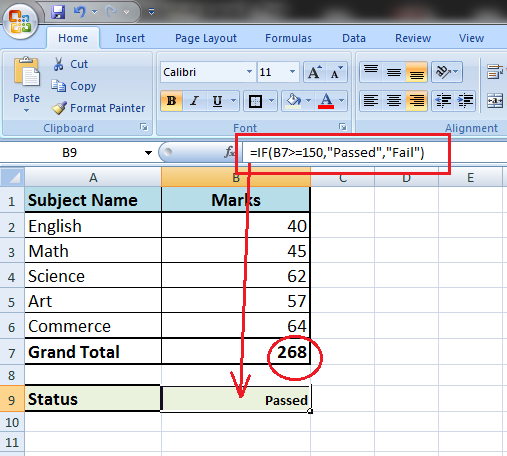Now we taken the marks of another student as shown under-

The total marks are below 150 so the result is “Failed”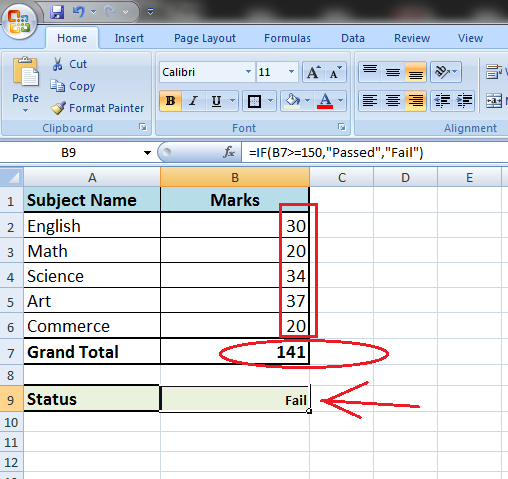# Example No. 4 for “If- Else” Formula in Excel :

Here in this example we need the stock status in an office.

The Condition is – if Cell “A2” is iPhone and Cell “B2” is 64 GB then result will be “Available” otherwise result will show “Out of Stock”.

=IF(AND(A2=”iPhone”,B2=”64GB”),”Available”,”Out of Stock”)

We need to paste this formula in every cell of column “C” to get the result of every cell of our data.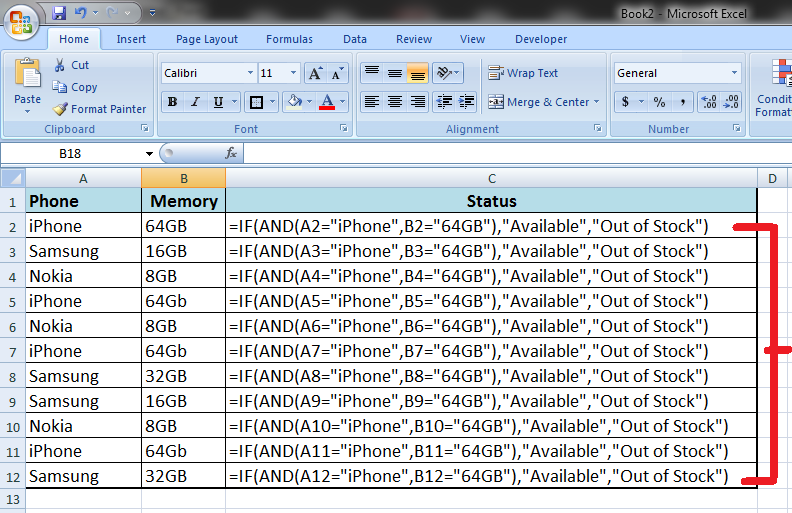So, above is the formula to get the result if “iPhone 64GB” is the combination of cells the result is “Available” and if not then result is “Out of Stock”.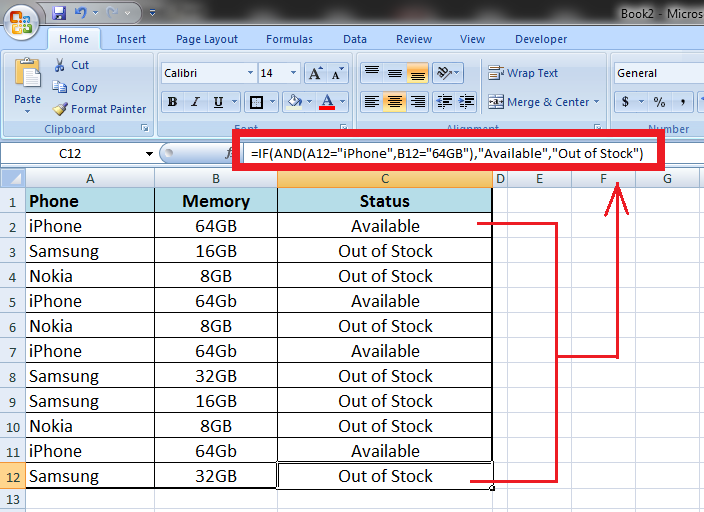Friends above are some example of “If, Else” Formula in MS excel we will update more “If, else example to make you better understand “if, else” function.

If you have any question or suggestion, please comment in the comment box we will solve your problem in our next article.

### 1 thought on ““If, Else” Formula in Excel with 4 Examples (Very Easy)”

1.Sir, I am submitting one Excel situation and work to do as under.

Situation.A) In sheet 1 i want to type names of students. Few have done homework, few have not done homework and few have incomplete homework.
B) There are three other worksheets namely 1)Homework Done, 2) Homework not done, 3) Homework incomplete.

Work:- 1) In sheet 1, I want to fill names of the students with their homework situation (done/not done/incomplete).
2) But as i fill names in sheet 1 i want their names (Row) auto filled in concerned sheets.

As i am new to Excel, I hope i have managed to say what i want to do.

Sir, kindly guide me through Video.